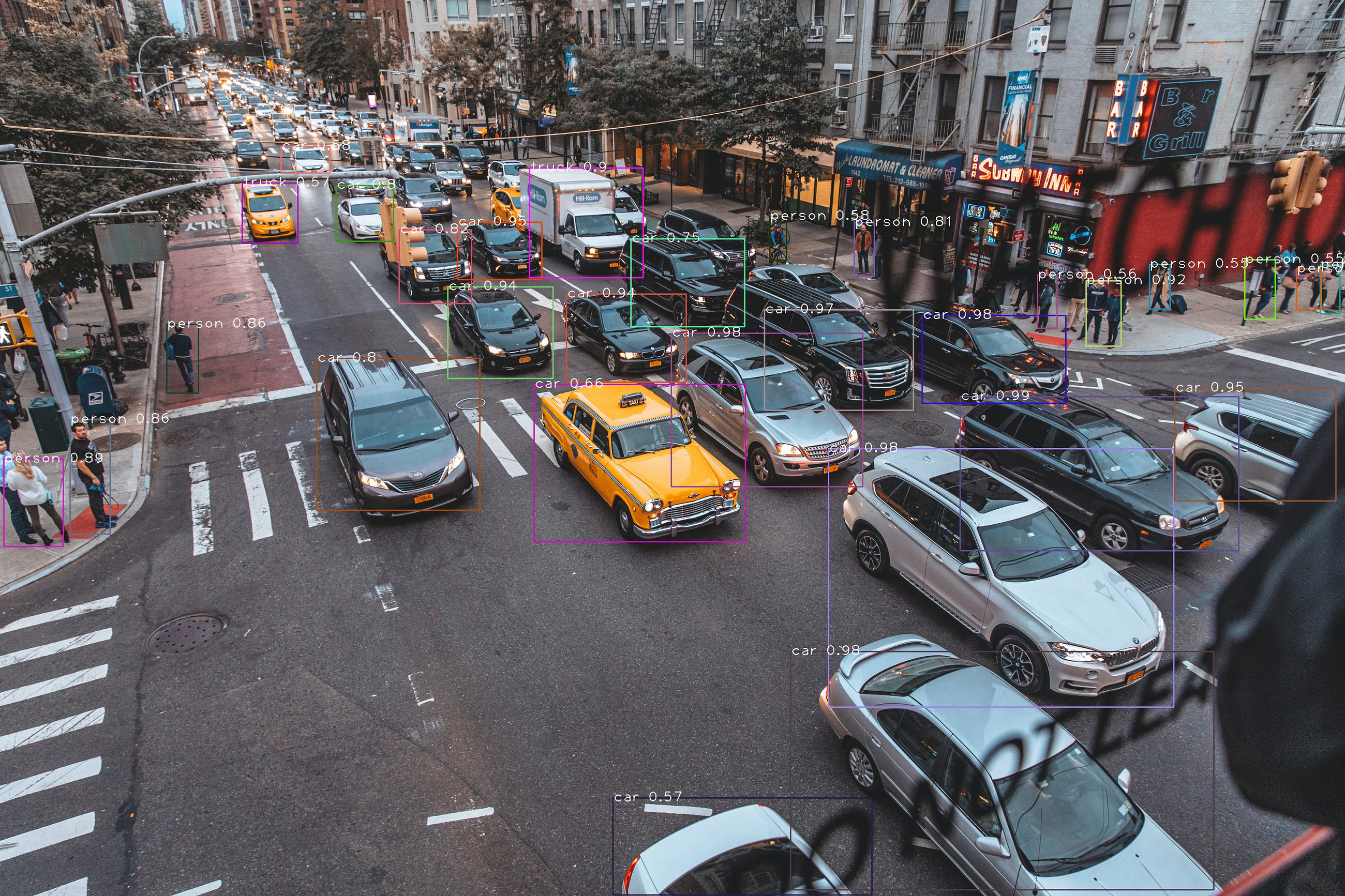# Object Detection Using You Look Only Once (YOLO)# Object Detection Using You Look Only Once (YOLO)

What is YOLO?

Object detection is one of the classical problems in computer vision where you work to recognize what and where. The problem of object detection is more complex than classification, which also can recognize objects but doesn’t indicate where the object is located in the image.

YOLO is a convolutional neural network (CNN) for doing object detection in real-time. The algorithm applies a single neural network to the full image, and then divides the image into regions and predicts bounding boxes and probabilities for each region. These bounding boxes are weighted by the predicted probabilities.

Let’s see how to use yolo pretrained model to detect the object. The Pretrained models are trained with 80 types of classes. you need to download the pretrained model weights and configuration file of the specific models here and download the coco- namefile. I am going to use YOLOv3–416 pretrained model weights

Yolo can be applied to

1. Image
2. Video
3. Webcam

Import the dependencies

`import cv2import numpy as np`

Read the model weights and the configuration file

`net = cv2.dnn.readNet('yolov3.weights' , 'yolov3.cfg')`

Create a list of classes by reading from the coco.names file

`classes = []with open('coco.names' , 'r') as f:    classes = f.read().splitlines()`

`image = cv2.imread(file)height , width , _ = image.shape`

here the converting the image to specfic form by that we can pass this as input to the model

`blob = cv2.dnn.blobFromImage(image , 1/255 , (416,416) ,(0,0,0) , swapRB = True , crop = False)`

Prediction , while predicting in the output layer will get about 85 parameters first four are bounding boxes x,y,h,w and fifth one is confidence and remaining are the classes

`net.setInput(blob)output_layer_names = net.getUnconnectedOutLayersNames()layeroutputs = net.forward(output_layer_names)`

Extracting the bounding boxes, confidence and classes of the object

`boxes=[]confidences=[]class_id=[]for output in layeroutputs:    for detection in output:        score = detection[5:]        ids = np.argmax(score)        confidence = score[ids]        if confidence > 0.5:             center_x = int(detection*width)# to denoramalize multiplying with original h and w            center_y = int(detection*height)            w = int(detection*width)            h = int(detection*height)            x = int(center_x - w/2)            y = int(center_y - h/2)            boxes.append([x,y,w,h])            confidences.append(float(confidence))            class_id.append(ids)`

To avoid many boxes we are picking up the boxes with high confidence

`indexes = cv2.dnn.NMSBoxes(boxes,confidences ,0.5,0.4)`

Plotting the bounding boxes on the image

`font = cv2.FONT_HERSHEY_PLAINcolors = np.random.uniform(0,255,size=(len(boxes), 3))if len(indexes)>0:    for i in indexes.flatten():        x,y,w,h = boxes[i]        label = str(classes[class_id[i]])        confi = str(round(confidences[i],2))        color = colors[i]        cv2.rectangle(image , (x,y) , (x+w,y+h) , color ,5)        cv2.putText(image , label +" "+confi , (x,y+20) ,font ,5 ,(255,255,255),3)cv2.imshow("Frame" , image)cv2.imwrite('output.jpg',image)cv2.waitKey(0)cv2.destroyAllWindows()`